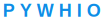# CP05数据格式归一化

excel 是现代报表应用中非常重要的一种数据格式，要进行报表处理，不可避免的要应用到 excel 数据的读取操作，所以今天我们就来看看，如何进行相关的数据处理吧。这也是进入报表世界的一个非常重要的基本功。

# 5 数据格式归一化-字段合并

## 5.1 用例故事1.X-Y： 空间坐标数据，他是来自于两个字段 Xserial，Yserial，通过这两个字段进行字符串拼接而来。 其中 Xserial 代表横坐标，Yserial 代表纵坐标。

2.低温采样点占比： 就是计算低温采样点数/采样时刻数，这样就可以估计出一天之内测量到低温的大致情况。

### 5.1.1 用例描述

1.用户输入期待处理的文件路径，输出结果文件的，相关的信息结构

[ 输入文件绝对路径，输出文件绝对路径]

1. 程序读取 grid.xlsx 文件，转换为内存中的中间流，成为一个 Data set 的格式。

3.程序根据入参中“输出文件绝对路径”，创建一个输出结果 grid-geo.xlsx 文件的句柄。；

4.应用程序对 Data set 的表头进行扩展，增加两列，名字分别为

5.应用程序对内存中的 Data set 的行数据，进行逐行处理。

5.1 应用程序定位到列“Xserial“，和”Yserial“, 分别识别出其中对于的字符串， 将 字符串 1（key=Xserial）和字符串 2（key=Yserial）进行拼接得到字符串 3=字符串 1（key=Xserial）-字符串 2（key=Yserial）。

5.2 将拼接的字符串 3,赋值给新增加字段 X-Y；

5.3 应用程序定位到列“全天采样时刻数“，以及”低温采样数“，提取其中的字符串，将字符串转换为数字类型。（整型）

5.4 应用程序计算 int1（key=低温采用数）/int2(key= 全天采样时刻数)，计算结果存入 int3= int1/int2;

5.5 应用程序将新计算的 int3，赋值给新增加字段“低温采样占比“

1. 一行处理完毕以后，顺序处理后续行的数据。

7.全部数据处理完毕以后，实现对 X-Y 列，和“低温采样占比列“的赋值操作。

8.应用程序将内存中的 data set 写入输出结果 xlsx 文件的句柄， 相关文件决对路径来自输入数据。

举例：D:\PAE\ grid-geo.xlsx

9.应用程序返回程序执行响应 [ 执行结果，输出结果文件信息]

D:\PAE\ D:\PAE\ grid-geo.xlsx

### 5.1.2 输入数据准备### 5.1.3 IPO 分析

#### 5.1.3.1 输入数据分析（I）

[ 输入文件绝对路径，输出文件绝对路径]

#### 5.1.3.2 处理过程（P）

1. 将 Xserial（（栅格 X 方向编号）、Yserial（栅格 Y 向编号），按 Xserial- Yserial 的形式，生成新字段名为“栅络 XY 编号”。如 Xserial 为 11611，Yserial 为 73318，新生成字段“栅络 XY 编号”为 11611-73318。合并时需考虑数据格式。

2. 计算字段：低温采样点占比。

2.1 应用程序定位到列“全天采样时刻数“，以及”低温采样数“，提取其中的字符串，将字符串转换为数字类型。（整型）

2.2 应用程序计算 int1（key=低温采用数）/int2(key= 全天采样时刻数)，计算结果存入 int3= int1/int2;

2.3 应用程序将新计算的 int3，赋值给新增加字段“低温采样占比“

### 5.1.4 输出数据（O）

D:\PAE\ D:\PAE\ grid-geo.xlsx

### 5.1.5 用例约束

1、 请使用 python3 及以上版本

2、 请使用 os 组件

3、 请使用字符串完成拼接

4、 Xy 数据合并请考虑兼容多种数据格式转换

## 5.2 设计方案简介

### 5.2.1 设计要点

Excel 组件的应用

``````xlrd.book.ensure_unicode='utf-8' #设置编码

data=xlrd.open_workbook(file_path) #打开文件

sheet_names = data.sheet_names() #获取文件中所包含的 Sheet 名

table = data.sheet_by_index(0)

rows_count = table.nrows #取总行数

cols_count = table.ncols #取总列数

col_data = table.col_values(0) #取第一列的数据
``````

### 5.2.2 调用关系### 5.2.3 流转换

1、rows_count = table.nrows #取总行数2、列表生成式+字典生成式的混合运用

``````[ dict(zip(row_head,table.row_values(index)) )for index in range(1, rows_count) ]
``````3、for row,i in enumerate(file_data) :

print( row,i) 遍历list得到字典：4、使用 dict-hash 修改数据：

``````i["X-Y"]=str(i["Xserial"])+"-"+str(i["Yserial"])

i["低温采样占比列"]= str(round(int(i["低温采样数"])/int(i["采样时刻数"]), 2)\*100)+"%"
``````5、写入文件，应用二维数组写入print(row, loc, data)流穿越：5、生成文件：## 5.3 设计方案详解

### 5.3.1 领域对象模型

#### 5.3.1.1 Excel 对象装载#### 5.3.1.2 流转换及输出### 5.3.2 关键技能点### 5.3.3 excle 组件 xlwt

#### 5.3.3.2 语法说明

``````import xlwt
# 创建一个workbook 设置编码
workbook = xlwt.Workbook(encoding = 'utf-8')
# 创建一个worksheet

# 写入excel
# 参数对应 行, 列, 值
worksheet.write(1,0, label = 'this is test')

# 保存
workbook.save('Excel_test.xls')``````

#### 5.3.3.3 Case 举例

``````workbook = xlwt.Workbook(encoding = 'ascii')
style = xlwt.XFStyle() # 初始化样式
font = xlwt.Font() # 为样式创建字体
font.name = 'Times New Roman'
font.bold = True # 黑体
font.underline = True # 下划线
font.italic = True # 斜体字
style.font = font # 设定样式
worksheet.write(0, 0, 'Unformatted value') # 不带样式的写入

worksheet.write(1, 0, 'Formatted value', style) # 带样式的写入

workbook.save('formatting.xls') # 保存文件``````### 5.3.4 excle 组件 xlrd

#### 5.3.4.2 语法说明

1）获取book中一个工作表

``````table = data.sheets() #通过索引顺序获取

table = data.sheet_by_index(sheet_indx)) #通过索引顺序获取

table = data.sheet_by_name(sheet_name)#通过名称获取
``````

``````names = data.sheet_names() #返回 book 中所有工作表的名字

data.sheet_loaded(sheet_name or indx) # 检查某个 sheet 是否导入完毕
``````2）行的操作

``````nrows = table.nrows #获取该 sheet 中的有效行数

table.row(rowx) #返回由该行中所有的单元格对象组成的列表

table.row_slice(rowx) #返回由该列中所有的单元格对象组成的列表

table.row_types(rowx, start_colx=0, end_colx=None) #返回由该行中所有单元格的数据类型组成的列表

table.row_values(rowx, start_colx=0, end_colx=None) #返回由该行中所有单元格的数据组成的列表

table.row_len(rowx) #返回该列的有效单元格长度
``````3）列(colnum)的操作

``````ncols = table.ncols #获取列表的有效列数

table.col(colx, start_rowx=0, end_rowx=None) #返回由该列中所有的单元格对象组成的列表

table.col_slice(colx, start_rowx=0, end_rowx=None) #返回由该列中所有的单元格对象组成的列表

table.col_types(colx, start_rowx=0, end_rowx=None) #返回由该列中所有单元格的数据类型组成的列表

table.col_values(colx, start_rowx=0, end_rowx=None) #返回由该列中所有单元格的数据组成的列表
``````4）单元格的操作

``````table.cell(rowx,colx) #返回单元格对象

table.cell_type(rowx,colx) #返回单元格中的数据类型

table.cell_value(rowx,colx) #返回单元格中的数据

table.cell_xf_index(rowx, colx)
``````

♦ 单元格：单元格是表格中行与列的交叉部分，它是组成表格的最小单位，可拆分或者合并。单个数据的输入和修改都是在单元格中进行的### 5.3.5.1 应用目的

#### 5.3.5.2 语法说明

1、导入模块

``````import xlrd

import xlutils.copy
``````

2、打开模块表

``````book = xlrd.open_workbook('test.xls', formatting_info=True)
``````

3、复制模块表

``````wtbook = xlutils.copy.copy(book)

wtsheet = wtbook.get_sheet(0)
``````

4、写入模块表

``````wtsheet.write(0, 0, 'Ok, changed!')
``````

5、保存模块表

``````wtbook.save('test.xls')
``````

#调用 xlrd.open_workbook()时，如果不指定 formatting_info=True，那么修改后整个文档的样式会丢失。对一个单元格进行 write 操作时，如果不指定样式，也会将原来的样式丢失。

#### 5.3.5.3 Case 举例

``````import xlrd

import xlutils.copy

import os

def write_Excel(r, c, msg):

"""

:param r: 坐标行

:param c: 坐标列

:param msg: 写入信息

:return:

"""

file_path = os.path.abspath(os.path.join(os.path.dirname(**file**), 'test.xls'))

book = xlrd.open_workbook(file_path, formatting_info=True) # 读取 Excel
``````

# 复制表

``````copy_book = xlutils.copy.copy(book)

copy_sheet = copy_book.get_sheet(0)
``````

# 指定单元格写入信息

``````copy_sheet.write(r, c, msg)
``````

# 保存文件

``````copy_book.save(file_path)

if __name__ == '__main__':
msg = 'test'
``````

# （2，3）为 D3 单元格

write_Excel(2, 3, msg)#### 5.3.5.4 应用注意点

· open_workbook()内若没有添加 formatting_info=True，则保存的文件不会保留原有格式；

· Excel 文件打开状态下，不可 xlutils 读取复制。运行会报错；

· 最新版本 xlrd 已不支持读取 xlsx 文件，运行也会报错。

### 5.3.6 excle 组件 openpyxl 库

#### 5.3.6.1 应用目的

Python 处理 excel 的第三方库有：xlrd，xlwt，openpyxl等。

#### 5.3.6.2 语法说明

``pip install openpyxl``

``pip install openpyxl -i https://pypi.tuna.tsinghua.edu.cn/simple``

Excel 基本概念

•工作簿(workbook)：一个 Excel 文档；

•工作表(sheet)：一个工作簿可以包含多个表, 如：sheet1， sheet2 等;

•列(column): 列地址是从 A 开始的;

•行(row): 行地址是从 1 开始的;•单元格(cell)：指定行和列的格；

Excel 操作

Excel 不管读写都是“三板斧”：加载 workbook，打开 sheet，操作 cell1.首先导入模块：

``from openpyxl import load_workbook``

2.加载工作簿：

#### 可以使用相对路径也可使用绝对路径

``````wb = load_workbook("学生表.xlsx")

3.打开工作表

``````#打开工作表两种方式：
#方式一：通过工作表名称打开工作表
sheet=wb["sheet1"]
#方式二：获取活跃的工作表
sheet=wb.active    #['sheet1']``````

#获取所有的工作表

wb.sheetnames

#[‘sheet1’]#修改工作表名称

sheet.title=“students”

#获取工作表名称

sheet.title

#students

4.单元格常用操作

``````sheet.cell(2,3)    #<Cell 'students'.C2>
sheet["C2"]    #<Cell 'students'.C2>``````

#方式一 sheet.cell(2,3).value #60

#方式二 sheet[“C2”].value #60

#方式一 sheet.cell(2,4).value=“及格”

#方式二 sheet[“D3”]=“及格”

#方式三 sheet.cell(4,4,“良好”)

#保存工作簿 wb.save(“学生表.xlsx”)#获取最大行：sheet.max_row #8

#获取最大列：sheet.max_column #4

#获取A1A3**的单元格cell1=sheet[‘A1:A3’]

#**获取第一行和第二行的单元格cell2=sheet[1:2]

#获取A列到C**列的单元格cell3=sheet[‘A:C’]

``````方法二：iter_rows 和 iter_cols 方法，此方法得到的是一个可迭代序列
#获取行数1-2且列数1-4的单元格，逐行读取cell4=sheet.iter_rows(min_row=1, max_row=2, min_col=1, max_col=4)for cell in cell4:    print(cell)
# 上面获取的是单元格，如果想得到单元格的值，只需加个参数cell4=sheet.iter_rows(min_row=1, max_row=2, min_col=1, max_col=4,values_only=True)
#获取列数1-4且行数1-2的单元格，逐列读取cell5=sheet.iter_cols(min_col=1, max_col=4,min_row=1, max_row=2)for cell in cell5:    print(cell)
#同样，想获取单元格的值，加参数cell5=sheet.iter_cols(min_col=1, max_col=4,min_row=1, max_row=2,values_only=True)``````

5.插入/删除行、列#第 2 行开始删除 3 行 sheet.delete_rows(idx=2,amount=3)#插入列 sheet.insert_cols(idx=数字编号,amount=要插入列数)#删除列 sheet.delete_cols(idx=数字编号,amount=要插入列数)``from openpyxl import load_workbook# 生成折线图from openpyxl.chart import LineChart,Referencewb=load_workbook("销售数据.xlsx")sheet=wb.activechart=LineChart()# 图的标题chart.title="手机销售数据统计"# y轴标题chart.y_axis.title="销量（单位：万台）"# x轴标题chart.x_axis.title="季度"# 数据来源data=Reference(worksheet=sheet,min_row=2,max_row=4,min_col=1,max_col=5)# 设定X轴项目名称,项目名称来自第一行的第二列至第五行categories=Reference(sheet,min_col=2,min_row=1,max_col=5,max_row=1)# 给折线图添加数据，数据源中有系列名称，系列名称来自行，第一行为系列名称chart.add_data(data,from_rows=True,titles_from_data=True)chart.set_categories(categories)sheet.add_chart(chart,"B6")wb.save("销售数据.xlsx")``
``from openpyxl import load_workbook#生成柱状图from openpyxl.chart import BarChart,Referencewb=load_workbook("销售数据.xlsx")sheet=wb.activebc=BarChart()# 图的标题bc.title="手机销售数据统计"# y轴标题bc.y_axis.title="销量（单位：万台）"# x轴标题bc.x_axis.title="季度"# 数据来源bc_data=Reference(worksheet=sheet,min_row=2,max_row=4,min_col=1,max_col=5)# 设定X轴项目名称,项目名称来自第一行的第二列至第五行bc_cat=Reference(sheet,min_col=2,min_row=1,max_col=5,max_row=1)# 给柱状图添加数据，数据源中有系列名称，系列名称来自行，第一行为系列名称bc.add_data(bc_data,from_rows=True,titles_from_data=True)bc.set_categories(bc_cat)sheet.add_chart(bc,"B6")wb.save("销售数据.xlsx")``

1.3.6.3 应用注意点

Starter
MicroServ
Tutorials
Report
Blog